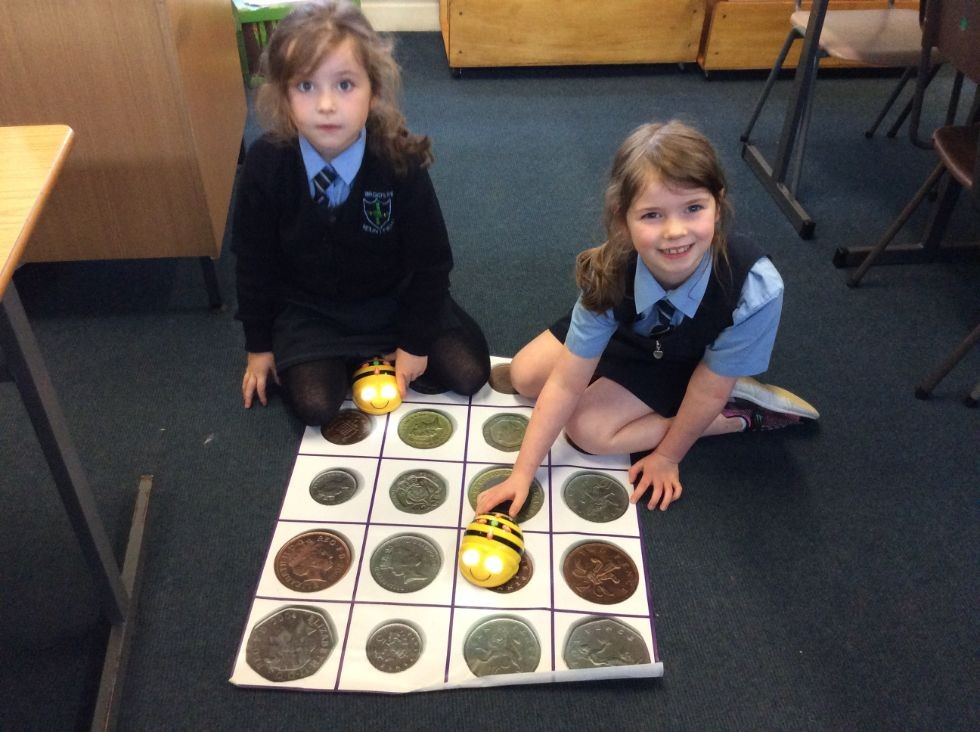## Numeracy## Mathematics

Using Mathematics Using Mathematics describes the confidence and ability to apply mathematical skills in a range of meaningful contexts.  Standards of pupil competency in Mathematics and Numeracy are assessed through the cross-curricular skill of Using Mathematics.

Across the curriculum, at a level appropriate to their ability, pupils should be enabled to:

• choose the appropriate materials, equipment and mathematics to use in a particular situation
• use mathematical knowledge and concepts accurately
• work systematically and check their work
• use mathematics to solve problems and make decisions
• develop methods and strategies, including mental mathematics
• explore ideas, make and test predictions and think creatively
• identify and collect information
• read, interpret, organise and present information in mathematical formats
• use mathematical understanding and language to ask and answer questions, talk about and discuss ideas and explain ways of working
• develop financial capability;
• use ICT to solve problems and/or present their work.